Bottom line: In this Excel formula challenge you will learn how to write a formula to find the first date for each month in a set of data.

Skill level: Intermediate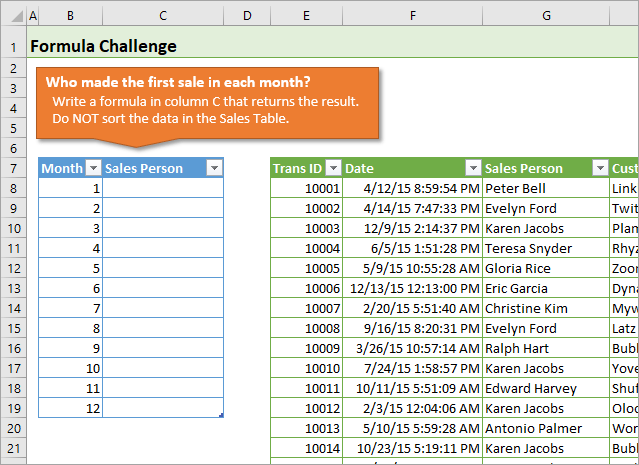## Video Explanation of the Challenge

Watch on YouTube and give it a thumbs up.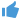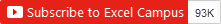## The Competition

Raylene, a member of The Ultimate Lookup Formulas Course, asked a great question about one of the assignments in the course.

For this challenge, the company's sales team has competition to see who can make the first sale of the month.  It's our job to create a report with the list of names for each month's winner.

This task is fairly easy if we can sort the data in the Sales Table.  But what if we can't sort it?  What would the formula look like then?

So, my challenge to you is to write a formula in column C to return the name of the sales person that made the first sale in each month.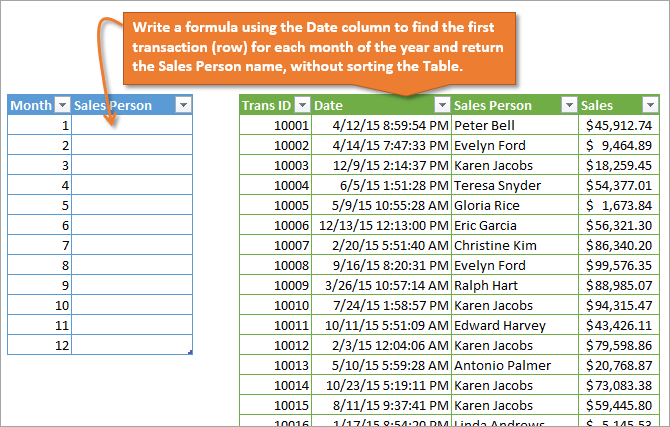Each row in the Sales Table is a transaction.  The Date column (column F) contains the transaction date.  Use the Date column to find the first sale for each month of the year, and return the sales person's name from column G.

The only rule is that you cannot sort the Sales Table.

The following image shows the results you are trying to produce.  Each name is the first person to make a sale in the month.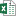Formula Challenge - First Sale Lookup.xlsx (63.0 KB)Formula Challenge - First Sale Lookup - Solutions.xlsx (553.1 KB)Formula Challenge - First Sale Lookup - Solutions - Regular References.xlsx (452.1 KB)

The file uses Excel Tables.  If you're not familiar with Excel Tables yet, you can turn off the structured reference Table formulas, and complete the challenge with regular formula references.

I added a solution file above that uses regular range references (\$H\$8:\$H\$1007) instead of structured reference Table formulas (tblSales[Date]).  You can use that file if you are not familiar with Table formulas yet.

## The Solution Videos

The videos below contain explanations for some of the most popular solutions that were submitted in the comments.  The solution file can be downloaded in the section above.

I want to say a big THANK YOU to everyone that participated.  We all learned a lot from your solutions.

Here's a link to the playlist of the videos on YouTube if you'd prefer to watch them there.

### Video #1: Array Formulas Explained

The most popular solution was using a MIN IF array formula combined with VLOOKUP or INDEX MATCH.  In this first video I explain the basics of an array formula.  I step through how they calculate on a range of values, and how to use Ctrl+Shift+Enter to enter the formula in Excel.

Watch on YouTube and give it a thumbs up.### Video #2: VLOOKUP or INDEX MATCH with MIN IF Array

In this video you will learn how to combine the MIN IF array formula with either VLOOKUP or INDEX MATCH to return the name of the salesperson.

Both techniques return the same result.  However, INDEX MATCH is less prone to errors when columns are added/deleted.  We can also use INDEX MATCH to return a value to the left of the Date column.

Checkout my free 3-part video series on the lookup formulas to learn more about these awesome functions.

Watch on YouTube and give it a thumbs up.### Video #3: The AGGREGATE Function

The AGGREGATE function is a great non-array solution.  That means we do NOT have to use Ctrl+Shift+Enter when entering this formula.  This is great because most users don't know or understand how to modify array formulas.

For this technique we use the Small function in AGGREGATE to return the smallest value.  The Small function has an optional [k] argument that allows us to return the 2nd, 3rd, etc. place value.  This makes the formula more versatile if our sales competition gets new rules.

We combine AGGREGATE with VLOOKUP or INDEX MATCH to return the salesperson name.

The AGGREGATE function was introduced in Excel 2010 for Windows, and is available on all Windows and Mac versions after 2010.

Watch on YouTube and give it a thumbs up.### Video #4: The New MINIFS Function

The MINIFS function was introduced in Excel 2016 and allows us to calculate a min if based on multiple criteria WITHOUT an array formula.  We do NOT have to use Ctrl+Shift+Enter for MINIFS.

It's similar to a SUMIFS or COUNTIFS formula, and is very easy to write.  It's probably the easiest solution for this challenge.  However, MINIFS is only available on the latest versions of Excel 2016 and you will probably need to be on an Office 365 subscription to get it.

Watch on YouTube and give it a thumbs up.### Video #5: Bonus – AGGREGATE with Multiple Criteria

We can also use the same technique with AGGREGATE to calculate a min if based on multiple criteria.  This is still a non-array formula, meaning we do NOT have to use Ctrl+Shift+Enter.

Watch on YouTube and give it a thumbs up.### And the Winner Is…

Like everything in Excel, there are many ways to solve this problem.  This is a great learning experience that should help add a few new techniques to your formula toolbox.

Each solution comes with it's own pros & cons, which I explain in the videos above.  I like the AGGREGATE function because of it's availability and versatility.

It's a non-array formula, so we don't have to use Ctrl+Shift+Enter, which is another plus.  However, it's still an advanced formula that the average user hasn't seen before.  So it will require some explanation if other people are going to be using your file.

MINIFS is the easiest solution, but not widely available if you or your users are on an older version of Excel.

Also, don't scroll down and look at the other answers until you try it. 😉

Good luck! 🙂

•Sumon says:

Hi! Jon,
I have a problem in my excel sheet, I didn’t find the function “Aggregate” in my excel 2007. Please, help me on this problem.
Thank you

•Mario says:

Here is my formula for lookup in the tblSales excel table the first sale for each month and retrieve the sales persons name:

{=VLOOKUP((MIN(IF(MONTH(tblSales[Date])= [@Month],tblSales [Date]))),\$F\$8:\$I\$1007,2,0)}

Approach: locate the dates within each month and identified the minimum date/time serial number which correspond to the first sale for each month. Then retrieve the sales person name for minimum date/time serial number for each month.
I used the following formulas:

1. VLOOKUP( ) = provides the value (text) of the sales person with the first sale of the month;

2. MIN( ) = provides the minimum value among the dates identified in the If/Month formula for a the month. It also provides the lookup value (the first sales of the month=minimum value of serialnumber on the identified dates from If/Month function;

3. IF( ) = in combination with month function to locate specific dates for each month in the Date field;

4. MONTH( ) = used with an array (“Date” field) to provide the criteria to lookup the values in a specific month (1=January, 2=February etc).

Please note that after applying Conditional Formatting/Highlights Duplicates to the “Dates” field there are two first sales for April as follows:

1. Antonio Palmer – 4/1/2015 5:38:53 PM
2. Samuel Vasquez – 4/1/2015 5:38:53 PM

Thank you very much Jon this formula challenge was a great learning experience.

•Chuck says:

=VLOOKUP(MIN(IF(MONTH(tblSales[Date])=[@Month],tblSales[Date])),tblSales[[Date]:[Sales Person]],2,FALSE)
Using the VLOOKUP I was able to make it to work by using the lookup value as follow:
By comparing the MIN value of the TbaleSales Date = to the corresponding value in the tableFirstSale Month and comparing it against the tblSales Date and looking for the corresponding Sales Person within the array of tblSales Sales Person and picking the content of the column and row that matches the MIN date reflecting the Sales Person.
And tried to run and I got the result as requested.

I had started using first a formula that gave me only the first one but then decided to change apply the VLOOKUP instead. I pretty sure that there must be a better way but could not figure out expected the formula listed above, which did the work.

•Diskman Hsu says:

Hi Jon, after attempting to realize the video contents of this formula challenge, I seem to be incapable of catching up then learning the skills from what you want to present and teach at this section. I know the functions VLOOKUP, INDEX, MATCH ,MIN, IF…etc but have never seen the symbol “@” existing in a formula then certainly have no idea what it works for. May I know which section in your course that I should study ahead of going through this one? Thanks!

•Jon Acampora says:

Hi Diskman,
Great question. These are called structured references for Table formulas. As mentioned in video #2, you do not have to use structured references. Here is a video on a beginner’s guide to Excel Tables that explains more about structured reference formulas.

I also cover Tables and formulas in more depth in my Ultimate Lookup Formulas Course.

I hope that helps. Thanks!

•Jon Acampora says:

It might take a few minutes for the cache to clear before you see it there.

To answer your question about @, this notation is used in Table formulas to refer to a cell in the same Row as the formula. We can use the following notation to refer to a cell that is in a different column, but same row as the formula.

[@[Column Name]]

The cell that contains the formula will typically be in the same Table when we use notation like this. I’ll post a video on how to use Table formulas in the future.

Thanks again!

•John Lui says:

This is the formula I used to get the answer

{=INDEX(\$G\$8:\$G\$1007,MATCH(MIN(IF(MONTH(\$F\$8:\$F\$1007)=B8,\$F\$8:\$F\$1007)),\$F\$8:\$F\$1007,0),)}

•Denise says:

=IF(tblSales[@Date]>1/1/15,tblSales[@[Sales Person]],” “)

•Rolando Brabant says:

=INDEX(tblSales[Sales Person],MATCH(AGGREGATE(15,6,(tblSales[Date])/([@Month]=MONTH(tblSales[Date])),1),tblSales[Date],0))

•Bob says:

Hi Jon, I must say this problem is beyond my current skills. Searching the internet I found a few of the key components to build a solution but did not understand any of them thoroughly enough to use them effectively… The best I could come up with in the time I had to work on it was: =VLOOKUP(MIN(F\$8:F\$1007),F\$8:G\$1007,1,0) and of course it does not solve the problem. It is really great to see everyone’s replies. They help me pick up the missing pieces of the various solution options… I can honestly tell you that for any normal business situation I would have counted on the Sort feature. If the sort feature failed or I had a confidence problem with the sort results I would have spent no time attempting to write a formula to find them. I would have instead written a VBA sub that executed basic queries to get me to verifiable conclusions. Clearly learning the various formulas are awesome… Thank you and everyone that replied for all your perspectives and solutions…:-)

•Edil says:

Hi Jon,

I struggled quite a lot with this challenge and I did not succeed fully.
In stead of entering a large formula I figure that it would be best to set up some help columns. I use the Month nrs (Col A), to calculate in Col L (L8), the 1st of each month at midnight (day/time stamp):

=DATEVALUE(tblFirstSale[@Month]&”/1/15″)

For January this gives me: 1/1/15 12:00:00 AM
Then in Col M (M8), I use this info to calculated the closest larger date/tine stamp value (as this would be the first sale):

=SMALL(tblSales[Date],COUNTIF(tblSales[Date],”<”&L8)+1)

For January this gives me: 1/1/15 3:27:20 AM
Then in Col N (N8), I search for the row in the Date column where this date/time stamp value occurs:

=MATCH(M8,tblSales[Date],0)

For January this gives me: 447
Then in cell C8 I entered the following formula:

=INDEX(tblSales[Sales Person],N8)

The formula gave me “Teresa Snyder”, but as it is copied down to C9:C19, it doesn’t change, all cells in C8;C9 show “Teresa Snyder”. I didn’t manage to resolve this.

•Mandar says:

Create helper columns Month(H), Rank(I) and Month & Rank(J) in “tblSales” table.

Create helper column Month & Rank in “tblFirstSale” with Rank 1 for all the 12 rows.

“tblSales” table:

Rank=1+COUNTIFS([Date],”<"&G8,[Month],H8)+COUNTIFS(\$G\$8:G8,G8,\$H\$8:H8,H8)-1

"tblFirstSale" table:

Sales Person = VLOOKUP([Month & Rank],tblSales[[Month & Rank]:[Sales Person]],2,0)

•Jon Acampora says:

Hey Mandar,

Sorry, I didn’t see your reply. This is a great solution that does NOT require an array formula.

Mandar is using the COUNTIFS function to rank the dates for each day in the month, by counting how many days are less than the current date in each row of the sale table. That number is then concatenated with the month number to lookup the first date for each month, and return the sales person’s name.

I updated the solution file to include a sheet with your solution. The sheet is named COUNTIFS and it’s in the Non-Array Formulas section.

Thank you for posting this crafty solution! 🙂

•Alexis says:

I found that the most convenient way of to do this was using an array formula, as many already mentioned.

Here is the original French formula I used :

{=INDEX(tblSales[Sales Person]; EQUIV(MIN(SI(MOIS(tblSales[Date])=[@Month];tblSales[Date];””)); tblSales[Date];0))}

Witch should translate to this :

{=INDEX(tblSales[Sales Person], MATCH(MIN(IF(MONTH(tblSales[Date])=[@Month],tblSales[Date],””)), tblSales[Date],0))}

The core of this formula is to test if the month in the Date column matches the month listed in our result table. If so, it returns it’s own value, if not it returns nothing. At this point I tried to let this argument blank, but it would then returns FALSE or 0, witch didn’t allow the MIN function to work properly. Then, that MIN function within an array formula gives us the position of that smallest value. An Index/Match function is than used to find the matching Sales Person who did that first sale.

•alex ashin says:

array formula
=INDEX(tblSales[[Date]:[Sales Person]],MATCH(MIN(IF(MONTH(tblSales[Date])=B8,tblSales[Date],””)),tblSales[Date],0),2)

•VEDA says:

=VLOOKUP(MIN(IF(MONTH(tblSales[Date])=[@Month],tblSales[Date])),tblSales[[Date]:[Sales]],2,FALSE)

•surendra singh says:

apply month formula to sales table at any column and it will convert date column into month 1 to 12 after that apply index & match e.g. =INDEX(tblSales[Sales Person],MATCH([@Month],tblSales[Column1],0))

•Robert says:

This is my take Jon. It is an Array formula !
=MIN(IF(MONTH(tblSales[Date])=[@Month],tblSales[Date]))

•Asif says:

My simple array formula would be as below;
{=VLOOKUP([@Month],CHOOSE({1,2},MONTH(tblSales[Date]),tblSales[Sales Person]),2,0)}

•Maki says:

I suggest 2 formulas:

={INDEX(tblSales[Sales Person];MATCH(MIN(IF(MONTH(tblSales[Date])=[@Month];tblSales[Date]));tblSales[Date];0))}

or

={VLOOKUP(MIN(IF(MONTH(tblSales3[Date])=[@Month];tblSales3[Date]));tblSales3[[#All];[Date]:[Sales Person]];2;0)}

Thanks

•Daniel says:

Hi John,

My answer to this formula challenge is :

=INDEX(tblSales;EQUIV(MIN(SI((MOIS(tblSales[Date])=[@Month])*(tblSales[Date]””);tblSales[Date];9^9));tblSales[Date];0);3)

I try to translate this formula in english formula but i’m not sure.

=INDEX(tblSales;MATCH(MIN(IF((MOIS(tblSales[Date])=[@Month])*(tblSales[Date]””);tblSales[Date];9^9));tblSales[Date];0);3)

Regards

•sagar says:

Hi,

In month we have to give first Date (01/01/2015 like of each month
Next find Small date of every month once we get small date then we use Vlookup funcation for find that date sales Person name

={VLOOKUP(MIN(IF(tblSales[Date]>=[@Month],tblSales[Date])),tblSales[[Date]:[Sales Person]],2,FALSE)}

•MF says:

Here’s my formula attempt:

=INDEX(tblSales[Sales Person],MATCH(AGGREGATE(15,6,1/1/(TEXT(tblSales[Date],”MMM”)=TEXT([@Month]&”/1″,”MMM”))*tblSales[Date],1),tblSales[Date],0))

Indeed, we may use Pivot Table for this kind of question, without a single formula.
And the better thing is… we may get the result of “Tie” by using Pivot Table.

Did you know that both Antonio Palmer, Samuel Vasquez made the same transaction at the same time in April?

Happy Weekend!

•Jamil says:

The shortest NON-CSE Formula I came up with it this one.

=LOOKUP(2,1/(AGGREGATE(15,6,tblSales[Date]/((MONTH(tblSales[Date])=[@Month])*(tblSales[Date]>0)),1)=tblSales[Date]),tblSales[Sales Person])

•Jamil says:

Jon,

I noticed something in the source data. there are two identical date-time in April for the earliest date. So, which one of the below should be returned if both sales persons did the sale on the exact date, hour, minutes and seconds.

Trans ID Date Sales Person
10018 01 April 2015 5:38:53 PM Antonio Palmer
10023 01 April 2015 5:38:53 PM Samuel Vasquez

•michael says:

add a helper column k to fina month of each data: =MONTH(‘Formula Challenge’!\$F8)and copy down
then
INDEX(\$G\$8:\$H\$1008,MATCH(SMALL(IF(\$K\$8:\$K\$1008=\$B8,\$H\$8:\$H\$1008,””),1),\$H\$8:\$H\$1008,0),1)

•Mike excelisfun Girvin says:

Thanks for the cool post, Jon!

Maybe this formula with no Helper Column and no Array Operation:

=INDEX(tblSales[Sales Person],MATCH(MINIFS(tblSales[Date],tblSales[Date],”>=”&DATE(2015,[@Month],1),tblSales[Date],”<"&EOMONTH(DATE(2015,[@Month],1),0)+1),tblSales[Date],0))

•Peter says:

=INDEX(G:K;MATCH(COUNTIF(tblSales[Month];”<"&B8)+1;K:K;0);1), where two helper columns were inserted: column J(tblSales[Month])is for the month number from the date, and the column K is the serial number calculated from the Date column.

•Mike Fullard says:

=VLOOKUP(MIN(IF(MONTH(Date)=B8,Date)),tblSales[[Date]:[Sales Person]],2,FALSE)
Where “Date” is the range with all the dates. Use Ctl+Shift+Enter.

•Paul says:

Here the formula that I came up with. The formulas I used are Vlookup with the exact match and Minifs. I have set up the year in a cell by itself so that I can change the year. The date between 1-31 no matter what date it is.
Minifs finds the minimum date and time which Vlookup finds and give me the person name

=VLOOKUP(MINIFS(tblSales[Date],tblSales[Date],”>=”&DATE(\$C\$21,B8,1),tblSales[Date],”<"&DATE(2015,B8,31)),\$G\$8:\$H\$1007,2,0)

•excelisfun says:

I posted a solution, but the comment is not showing up!?!?

•Jon Acampora says:

Hey Mike! Sorry about that. The comment must be approved then takes awhile to clear the site cache… We’ll update the site to reflect that message. Thanks!

•Pablo says:

Hi Jon,
My solution is NOT an array formula, so no need of Shift+Ctrl+Enter.

=VLOOKUP(AGGREGATE(15,6,tblSales[Date]*1/(MONTH(tblSales[Date])=[@Month]),1),tblSales[[Date]:[Sales Person]],2,0)

It uses our familiar VLOOKUP (simpler and shorter than INDEX/MATCH duo) and to find the smallest entry by month, AGGREGATE comes to the rescue. Similar technique to Paul’s above, but with a simpler approach by using 1/array comparison, which eliminates the need to use it twice.
Agreed, Mike Girvin makes Excel fun and credit of the formula technique goes to him.

Thanks,
Pablo

•Britta says:

Not sure, if someone has already posted this version:
=+VLOOKUP(SMALL(tblSales[Date];[@Month]);tblSales[[Date]:[Sales Person]];2;0)

I used SMALL instead of MIN and for the kth-element I used the numbering of the table

•Adam Kopeć says:

CSE
=INDEX(tblSales[Sales Person];MATCH(SMALL(IF(MONTH(tblSales[Date])=[@Month];tblSales[Date]);1);tblSales[Date];0))

Power Query – but this using sorting 😉
let
Source = Excel.CurrentWorkbook(){[Name=”tblSales”]}[Content],
Sort = Table.Sort(Source,{{“Date”, Order.Ascending}}),
Month = Table.TransformColumns(Sort,{{“Date”, Date.Month, Int64.Type}}),
Duplicates = Table.Distinct(Month, {“Date”}),
Columns = Table.SelectColumns(Duplicates,{“Date”, “Sales Person”})
in
Columns

•Rajinder Singh says:

=+INDEX(tblSales[Sales Person],MATCH(MIN(IF(MONTH(tblSales[Date])=[@Month],tblSales[Date])),tblSales[Date],0))

Then ctrl+shift+enter

•Jan Malis says:

Hi John,
My knowledge of formulas is very poor but being your student in VBA-PRO course I sweated out some code to tackle the challenge.

in the cell: =EarliestSale([@Month])

in the VBA module:
Function EarliestSale(month1 As Integer)

Dim Person As String
Person = “nobody”
Dim monthStr As String
Dim location As Integer
Dim latestD As Date
latestD = “1.1.2016”

For i = 8 To 1007

location = InStr(Cells(i, 6).Value, “.”)
monthStr = Mid(Cells(i, 6).Value, location + 1, 2)
If InStr(monthStr, “.”) Then
monthStr = Left(monthStr, 1)
End If
Dim monthInt As Integer
monthInt = CInt(monthStr)
Debug.Print monthInt

If monthInt = month1 Then
If Cells(i, 6).Value < latestD Then
latestD = Cells(i, 6).Value
Person = Cells(i, 7).Value
End If
End If

Next i

EarliestSale = Person

End Function

p.s. my Czech Windows setting throws me date in our shape with days at the begining, so my code will probably need some modification to work in your setting ….

•Mike excelisfun Girvin says:

Maybe this one with no helper column or Array Operations:

=INDEX(tblSales[Sales Person],MATCH(MINIFS(tblSales[Date],tblSales[Date],”>=”&DATE(2015,[@Month],1),tblSales[Date],”<"&EOMONTH(DATE(2015,[@Month],1),0)+1),tblSales[Date],0))

•Maki S. Hussain says:

Hi,

1- To know the right sale person, we need to know the position of the lowest value of individual month. That can be done by match and index combination, but

2- To know the lowest value of individual month, I have used the array formula MIN(IF(MONTH(tblSales[Date])=[@Month];tblSales[Date]))

So, the complete formula became as shown below.

{=INDEX(tblSales[Sales Person];MATCH(MIN(IF(MONTH(tblSales[Date])=[@Month];tblSales[Date]));tblSales[Date];0))}

Do not forget to press CTRL-SHIFT-ENTER

•Paul Elliott says:

May not be the most elegant but here it goes

INDEX(tblSales[Sales Person],
MATCH(MIN(IF(VALUE(tblSales[Date])*(MONTH(VALUE(tblSales[Date]))=[@Month])0,
VALUE(tblSales[Date])*(MONTH(VALUE(tblSales[Date]))=[@Month]))),
tblSales[Date],0))

Basically multiply the Date column times the an array of 1’s and 0’s depending on whether the month matches. Then the Min If determines the smallest value that is not 0. From there, find that value in the date column with the MATCH function, and then return the corresponding value from the Sales Person column using the INDEX function.

•Alvin says:

will vlookup work for this problem?

•Ted Pettit says:

I’m afraid the solution I came up with isn’t unique but I went with the CSE route as well:
{=INDEX(tblSales[Sales Person],MATCH(SMALL(IF(MONTH(tblSales[Date])=[@Month],tblSales[Date],””),1),tblSales[Date],0)))}

•Cris says:

First I added a “month” column in tblSales (=MONTH([@Date]))

Then
=INDEX(tblSales,MATCH(MINIFS(tblSales[Date],tblSales[month],[@Month]),tblSales[Date],0),3)

Cris

•Mike says:

Looks like my array formula is same as others

=VLOOKUP(MIN(IF(MONTH(tblSales[Date])=[@Month],tblSales[Date])),tblSales[[Date]:[Sales Person]],2,FALSE)

CTRL-SHIFT-ENTER

•Amardeep says:

I have used this (to be entered as array through Ctrl Shift Enter). This can be modified to use INDEX instead of VLOOKUP if data layout so requires.

=VLOOKUP(MIN(IF(MONTH(tblSales[Date])=tblFirstSale[@Month],tblSales[Date],””)),tblSales[[Date]:[Sales Person]],2,0)

•AFSAL says:

={VLOOKUP(MIN(IF((MIN(IF(MONTH(tblSales[Date])=[@Month],\$F\$8:\$F\$1007,””)))=tblSales[Date],tblSales[Trans ID],””)),tblSales[[Trans ID]:[Sales Person]],3,0)}

First look at first sale person by time.
if any duplicate time came it will also look Transaction ID to get first sale person.

•Paul says:

=INDEX(tblSales[Sales Person],MATCH(AGGREGATE(15,6,(–(MONTH(tblSales[Date])=[@Month])*tblSales[Date]/–(MONTH(tblSales[Date])=[@Month])),1),tblSales[Date],0))

The key is the AGGREGATE function, which finds the earliest date for the current month.
AGGREGATE(15,6,(–(MONTH(tblSales[Date])=[@Month])*tblSales[Date]/–(MONTH(tblSales[Date])=[@Month]))

Using the AGGREGATE formula means you don’t need to enter this as an array formula.

The Aggregate expression has arguments 15, which uses SMALL and 6, which says ignore errors. The fourth argument,1, says get the smallest value from the array.

The array is: (–(MONTH(tblSales[Date])=[@Month])*tblSales[Date]/–(MONTH(tblSales[Date])=[@Month])),1)

The numerator gives dates in the current month and zeros otherwise. Since the AGGREGATE is looking for the smallest value, just using the numerator would give the result 0 for each month. So divide by the test that the month of the sale = current month, this will be zero for all dates but current months, giving #div0 values for all dates in the array not in the current month. These are ignored by the AGGREGATE function (due to second argument) so it will find the smallest (earliest) date from the current month.

Having found the earliest date in the current month, find its row number with the MATCH and then the corresponding sales name with INDEX>

Thanks to Mike Girvin (ExcelsFun) who demonstrated the technique in one of his excellent YouTube videos

Generic filters
Exact matches only
Filter by Custom Post TypeLearn 10 great Excel techniques that will wow your boss and make your co-workers say, "how did you do that??"